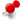total length measurement of an ellipseClassicListThreaded3 messagesOpen this post in threaded view
|

total length measurement of an ellipse

 I am trying to get the total length measured of an ellipse (or partial of one), and it doesn't appear to be reporting the correct length.  I create an ellipse in the following way: 1) select Ellipse Arc (Axis) 2) Click on origin (0,0) as center of ellipse. 3) Go up to 300mils to (0,300) to set endpoint of major axis. 4) Go to the right 100mils to (100,0) to set endpoint of minor axis. 5) Starting at 0 degree, end at 180 degrees to create a half ellipse. When I select the half ellipse, and measure Total Length of Selected Entities (and this ellipse is the only thing selected), I get a length of 711.0605mils.  Using some of the online calculators to calculate the circumference of an ellipse, using A=100mils (x-axis radius) and B=300mils (y-axis radius), the total circumference of the ellipse should be 1336.49mils.  Divided by 2 (since I have half an ellipse), I should get 668.25mils...NOT 711.061mils which LibreCAD is reporting.  Am I doing something wrong here?  I get the same wrong calculation for a full ellipse.  Changing dimensions doesn't seem to work, nor changing decimal settings or grid spacings (was thinking it was a rounding error).  If I use the same ellipse command, but create a circle (300mils major and minor axis), and measure the length, I get the correct response of 1884.96mils. Any suggestions?